# Extra for Experts: Maximal Linear System Response to a Digital Input

### Extra for Experts Maximal Linear System Response to a Digital Input

The remainder of this Chapter 1 shall fill with a theorem from linear system theory concerning the worst-case response of linear time-invariant systems when driven by digital signals. This theorem is useful for evaluating, among other things, worst-case crosstalk voltages and worst-case power supply noise voltages. The theorem begins with a rather abstract situation that reduces in the end to a more familiar digital situation.

The mathematical model underlying the theorem appears in Figure 1.5. This figure shows an ideal source, producing a waveform x ( t ) with an output limited to the range of ± A . No other requirements are placed upon x ( t ). It may have infinitely fast rise and fall times, it can transition at any time (not limited only to clock intervals), and it may attain intermediate voltages within the range of ±A. The signal x ( t ) is a superset of the class of all digital signals.

Figure 1.5. The maximum output from system H is bounded by equation [1.21]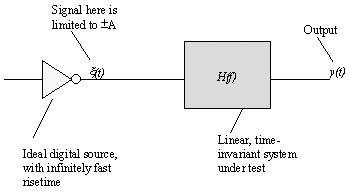The ideal source output is then presented to a linear, time-invariant system with transfer function H ( f ), corresponding impulse response h ( t ), and step response h ( t ), and step response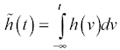. The output of the linear, time-invariant system is signal y ( t ).

The theorem states a bound Y on the amplitude of y ( t ) that holds for all possible signals x ( t ). Furthermore, this is a constructive theorem in that it also develops at least one particular function x ( t ) that is guaranteed to excite y ( t ) to its maximum value. The bound Y on the output amplitude is

Equation 1.21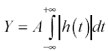One signal x ( t ) that will cause the bound to be reached is

Equation 1.22where the function sgn() is the sign function,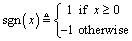The proof of this solution follows from an examination of the convolution integral for linear, time-invariant systems. The output y ( T ) at time T in response to any excitation is thus defined:

Equation 1.23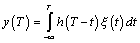If we wish to maximize the output at any particular time T , this integral grants us enormous flexibility to do so. We are free to assign whatever values to x ( t ) we choose, at every point t in time, provided that no value may fall outside the range ±A. Note that the various values of x ( t ) each are used but once inside the integral, with no complicating constraints. They may all be independently assigned. Therefore, the way to maximize the integral is simply to maximize the integrand for each value of t .

For example, the best choice for x ( ) would be whatever value maximizes its elemental contribution to the integral, h ( T “ 0 ) x ( ). If the algebraic sign of h ( T ) is positive, choose x ( ) = + A (the largest possible positive value). If the algebraic sign of h ( T ) is negative, choose x ( ) = “ A. That's all there is to it.

In general, the values for x ( t ) that maximize y at time T are x ( t ) = A sgn( h(T “ t )). In the special case where you wish to maximize the output at time T = 0, equation [1.22] results. Equation [1.21] results from inserting solution [1.22] into equation [1.23], evaluating the result at T = 0, and recognizing that h ( t ) sgn( h ( t )) = h ( t ).

Similar reasoning holds in the reverse algebraic direction. To minimize y , use x ( t ) = -A sgn( h ( T “ t )). For a linear system with symmetrical input (no DC offset), the worst-case positive and negative amplitudes are equal.

Let's extend this theorem to treat some special forms of impulse response. For example, what if the impulse response is everywhere positive? In that case the particular signal that maximizes the response is simply x ( t ) = A , a full-valued, steady-state input. This says that systems with purely positive impulse responses are special. In response to a bounded input, their outputs can never exceed the steady-state maximum output. In other words, they have no overshoot .

The condition h ( t )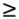0 may also be expressed in terms of the step response. The equivalent condition imposed on the step response is that the step response be monotonic. These three properties, therefore, always go together:

• Nonnegative impulse response
• Monotonic step response
• No overshoot in response to bounded input

What if the impulse response goes negative, but just for a short interval? That would place a dip in the step response, a short interval of nonmonotonic behavior. What happens to the maximum output signal in this case? I'd like to show you this answer and express it in terms directly observable on the step response waveform. To do so requires a little more calculus.

The answer may be found directly from equation [1.23], substituting our known solution for x ( t ), choosing to maximize the response at point T = 0, and substituting a new time variable u = - t .

Equation 1.24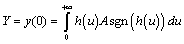Suppose now that you are given a list of the intervals of time [ pstart n , pend n ] over which h ( u ) were positive, and another list of intervals [ qstart m , qend m ] over which h ( u ) were known to be negative. You could then rewrite the integral as a sum of component integrals:

Equation 1.25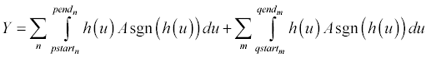By the construction of the p -intervals, the value of A sgn( h ( u )) within them is always A , so that factor may be pulled out from under the integral. Similarly, over all the q -intervals the value of A sgn( h ( u )) is “A , so that factor may be pulled out as well. The resulting expression looks like this:

Equation 1.26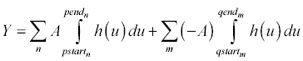Now you have a series of ordinary integrals of the impulse function of a linear system. Express these integrals in terms of differences between various points on the step response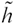:

Equation 1.27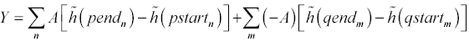If you have followed me so far, you will note that each of the terms within the left-hand summation is positive. Each of the terms within the rightmost summation is positive as well, by virtue of having been multiplied by “A . I can therefore eliminate all references to the sign of A by using the absolute value symbol:

Equation 1.28What this equation tells you to do is to first mark out all the maxima and minima on the step response. These are the points where the slope (the impulse response) changes sign. The take the absolute value of the successive differences between each of these extrema and add them up ( D 1 + D 2 + D 3 ...). Multiply by A and you have your answer. That's the biggest output you will ever see in response to a bounded input (Figure 1.6).

Figure 1.6. The response to a bounded excitation can grow larger than step response.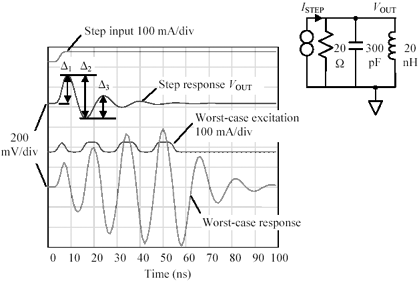Applying this theory to a real digital system takes a few more manipulations. First, we need to treat the issue of DC offset, because the voltages 0 and V CC , not ± A , generally bound a digital signal. Finally, we need to treat the issue of finite risetime.

The DC offset issue easily succumbs to a superposition analysis. To generate a solution, break the input signal into two components , a DC offset (V CC /2) and an AC signal (with amplitude A = V CC /2). Use your intuition to derive the DC steady-state output ( assuming the driver constantly put out V CC /2). Use the maximal value theorem [1.22] to tell you how much the output may range above or below the DC steady-state output. 

 This use of superposition works only for linear systems.

The mathematical model used to solve the finite-risetime part of the problem appears in Figure 1.7.

Figure 1.7. The magnitude of the largest possible response at y ( t ) is a function of the step response of the combined filter G ( f ) H ( f ).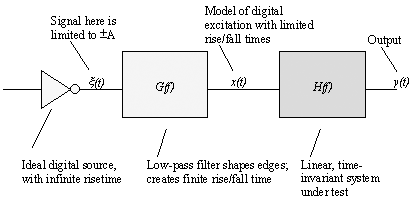Here the signal x ( t ) is put through a low-pass filter function G ( f ) whose purpose is to round off the (possibly) square edges of x ( t ) such that x ( t ) will never display a transition faster than that allowed by the step response of the filter G ( f ). For a suitable choice of G ( f ), the space of all signals available at x ( t ) includes pretty nearly all the things that could come out of a digital driver with rise and fall time t r . You will want to set up the filter G ( f ) to have a monotonic step response, and unity DC gain, in order that the excursion limits at x match those at x .

As a practical matter, what I do is make a step response measurement from the system under test using the system's own driver as the step source . The system driver therefore implements the function G ( f ) for me. I then mark off the extrema of the step response, measure the magnitudes of the inter-extreme excursions, sum their values, and divide by two (I measured the step response with a driver with range [0, V CC ] for which A = V CC /2; ergo, I scale the measured output by 1/2). The result tells me how much the output may range above or below the DC steady-state output.

This theorem applies to linear, time-invariant systems. It does not apply to limited, clamped, or nonlinear systems, or to systems that change significantly during the time within which the output is created.

It does, however, apply reasonably well to systems with nonlinear totem-pole drivers provided that the driver after each rising edge rapidly attains an output near V CC before the first reflections return from the end of the line. Under this condition the behavior of the driver during the time the signal reflects off the driver is indistinguishable from a simple, linear source connected to V CC , so the theorem applies. The same reasoning applies to drivers that rapidly pull low, attaining a near-zero state prior to the arrival of the first reflections from the far end of the line.

The space of candidate functions x used in the construction of the maximal solution includes all functions that slam back and forth between the given limits, without regard to the times at which they may do so. This space is a superset of the collection of all synchronous waveforms clocked at some fixed rate. Therefore, the upper bound Y is just that, an upper bound on the maximal excursion. It is possible in some situations that the maximal solution function x and its corresponding filtered version x cannot be produced by the target system and are therefore of little or no concern in worst-case analysis. This can happen in systems that have risetimes much shorter than the baud interval. In these cases it's a good idea to examine the waveform x so you can decide whether or not to worry about it.

In the design of high-speed LAN communications systems I have found this theorem to be of great value. In LAN systems the risetime and the baud interval are closely related , and the difference between the calculated Y and the actual, real-world upper bound is negligible.

This discussion highlights an important point about crosstalk in cable systems ”the long, lingering, diddling crosstalk signals that show up in response to a step input can, if excited with a worst-case input, easily be made to build up to significant voltages. Equation [1.28] predicts the worst-case crosstalk response from a single measurement of the step response of the system.

Equation [1.28] is also of great benefit in predicting the worst-case response of a power system to random surges of current. From a single measurement of the step response of a digital system ( swinging from minimum current to maximum current in one quick step) equation [1.28] predicts the worst-case power-supply excursion. This result will always meet or exceed the excursions computed based upon simulations of the power system response using sinusoidal or square-wave excitations.

POINT TO REMEMBER

• Resonance affects all physical structures, including bridges (Figure 1.8).

Figure 1.8. The Tacoma Narrows bridge collapsed on July 1, 1940.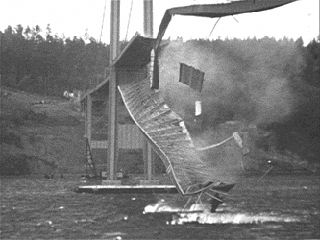For further study see: www.sigcon.comHigh-Speed Signal Propagation[c] Advanced Black Magic
ISBN: 013084408X
EAN: N/A
Year: 2005
Pages: 163